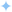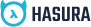Edit on GitHub

# Rank Functions

## Rank Functions in MySQL

The ranking functions in MySQL are used to rank each row of a partitioned part of a table.

These functions have to be used with the OVER() clause.

The ranking functions always assign a rank on the basis of the ORDER BY clause.

The rank is assigned to rows in a sequential manner.

The assignment of rank to rows always starts with 1 for a new partition.

There are 3 types of ranking functions supported in MySQL:

• dense_rank():

This function assigns a rank to each row within a partition. The ranks are assigned in a consecutive manner, if there two values are equal then they will be assigned the same rank, and the next rank value will be one greater than the previous rank assigned.

```SELECT subjects, s_name, mark, dense_rank()
OVER ( partition by subjects order by mark desc )
AS 'dense_rank'  FROM result;```
• rank():

This function assigns a rank to each row within a partition that has gaps. In this case, ranks are assigned in a non-consecutive manner i.e if there two records have the same value then they will be assigned the same rank, and the next rank value will be the previous rank plus the number of duplicates.

```SELECT subjects, s_name, mark, rank()
OVER ( partition by subjects order by mark desc )
AS 'rank' FROM result;```
• percent_rank():

This function returns the percentile rank of a row within a partition that ranges from zero to one. It shows the percentage of partition values lesser than the value in the current row, excluding the highest value in the partition.

```SELECT subjects, s_name, mark, percent_rank()
OVER ( partition by subjects order by mark )
AS 'percent_rank' FROM result;```
•Build apps and APIs 10x faster
•Built-in authorization and caching
•8x more performant than hand-rolled APIs